# Mike's Marbles

Mike wants to find 10 marbles. Mike finds 3 red marbles. Mike finds 3 blue marbles. Mike finds 3 green marbles. Does Mike find 10 marbles? Show and tell how you know.

Assessment

## Plan

#### Formal Mathematical Language and Symbolic Notation

A student may independently select a printed number line, number chart, ten frames, graph paper, etc. as they work on a task.

### Suggested materials

Engagement Image:

Teachers may project the image below to launch this task for their students, define nouns, promote discussion, access prior knowledge, and inspire engagement and problem solving.

## Solutions tabs

### Expert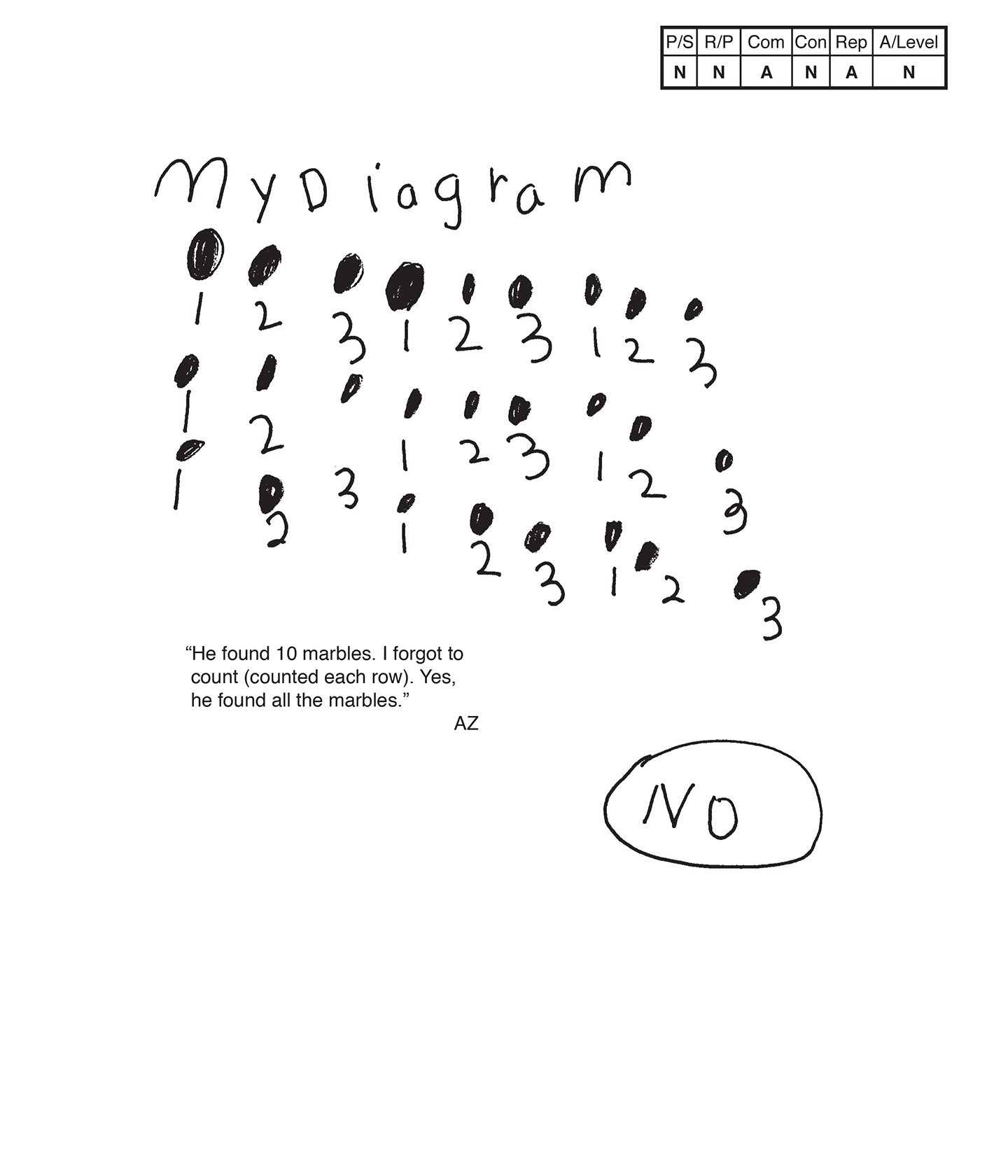This student does not meet the standard.

### Scoring Rationale

#### Novice

The student's strategy of diagramming nine red marbles, nine blue marbles, and eight green marbles does not work to solve this task. The student's answer, "No," their diagram, and scribing do not support the correct answer that Mike does not find 10 marbles.

#### Novice

The student's reasoning of making a set/group of nine red marbles, a set/group of nine blue marbles, and a set/ group of eight green marbles does not lead to a correct solution. The student does not demonstrate understanding of the underlying mathematical concept of combining three sets/groups of three different color marbles to determine if the total number of marbles is ten.

#### Apprentice

The student correctly uses the mathematical term diagram.

#### Novice

The student does not make a mathematically relevant observation about the underlying mathematics in the task.

#### Apprentice

The student attempts to make a diagram to represent Mike's marbles, but it is not accurate.

#### Novice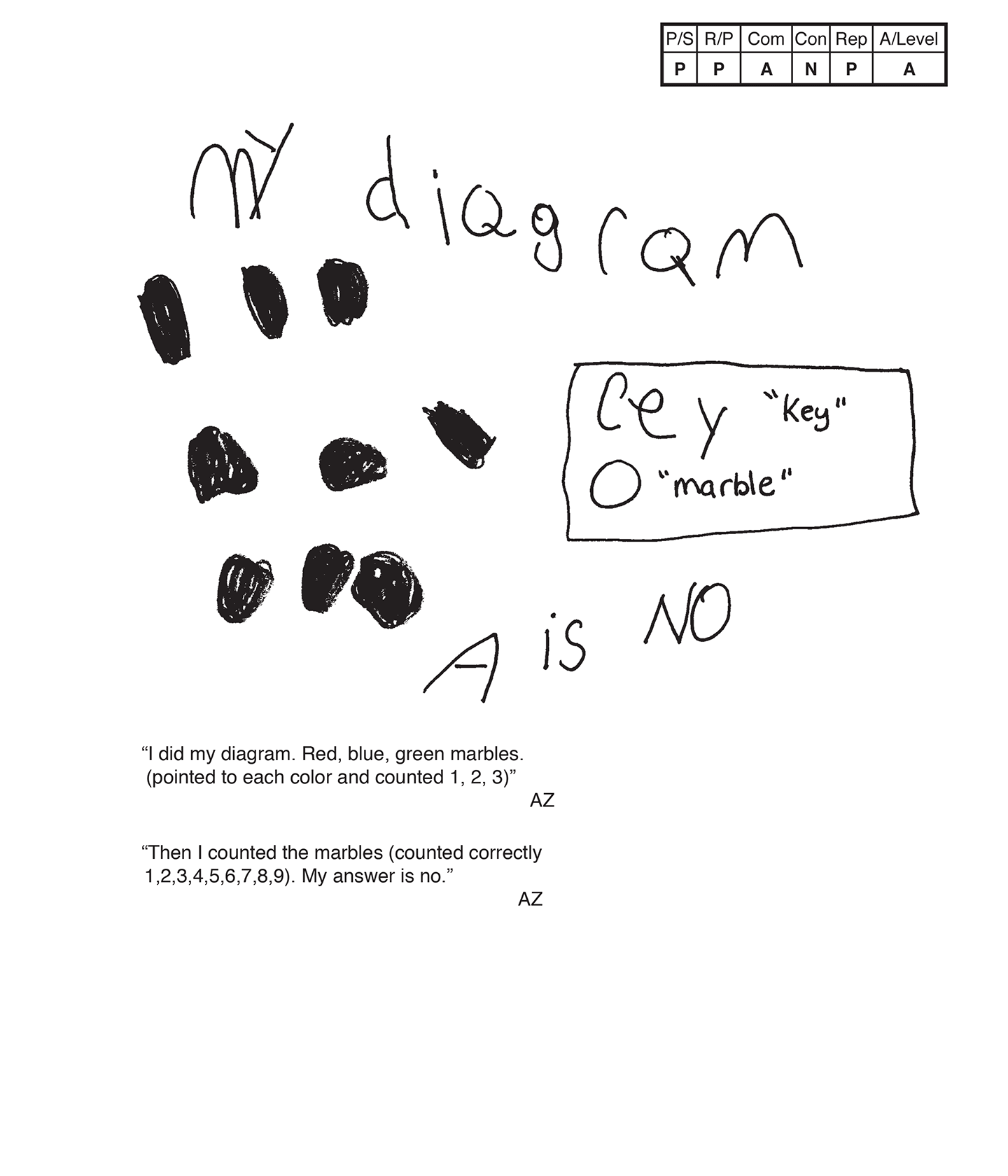This student does not meet the standard.

### Scoring Rationale

#### Practitioner

The student's strategy of diagramming three red marbles, three blue marbles, and three green marbles for a total of nine marbles and comparing to 10 marbles works to solve this task. The student's answer, "No," is correct, and the scribing supports their answer.

#### Practitioner

The student has a systematic approach and provides adequate mathematical basis to support their answer in their diagram and in the scribing.

#### Apprentice

The student correctly uses the mathematical term diagram. The student does not earn credit for the term "cey" (key). The student uses colored marbles in their diagram instead of a clear circle marble as indicated in the key.

#### Novice

The student solves the task but does not make a mathematically relevant observation about their solution.

#### Practitioner

The student's diagram is appropriate and accurate. The scribing provides the necessary label for the color of each marble.

#### Apprentice

Note:

The overall achievement level for this piece of student work falls under Exemplars exception to the rule category. If a student has all Apprentice scores or above, but a Novice in "Connections," the student may still receive an achievement level score of Apprentice. To learn more about Exemplars scoring, please refer to the section of your dashboard called "Tools for Success" and click on the link for “Using the Assessment Rubric.”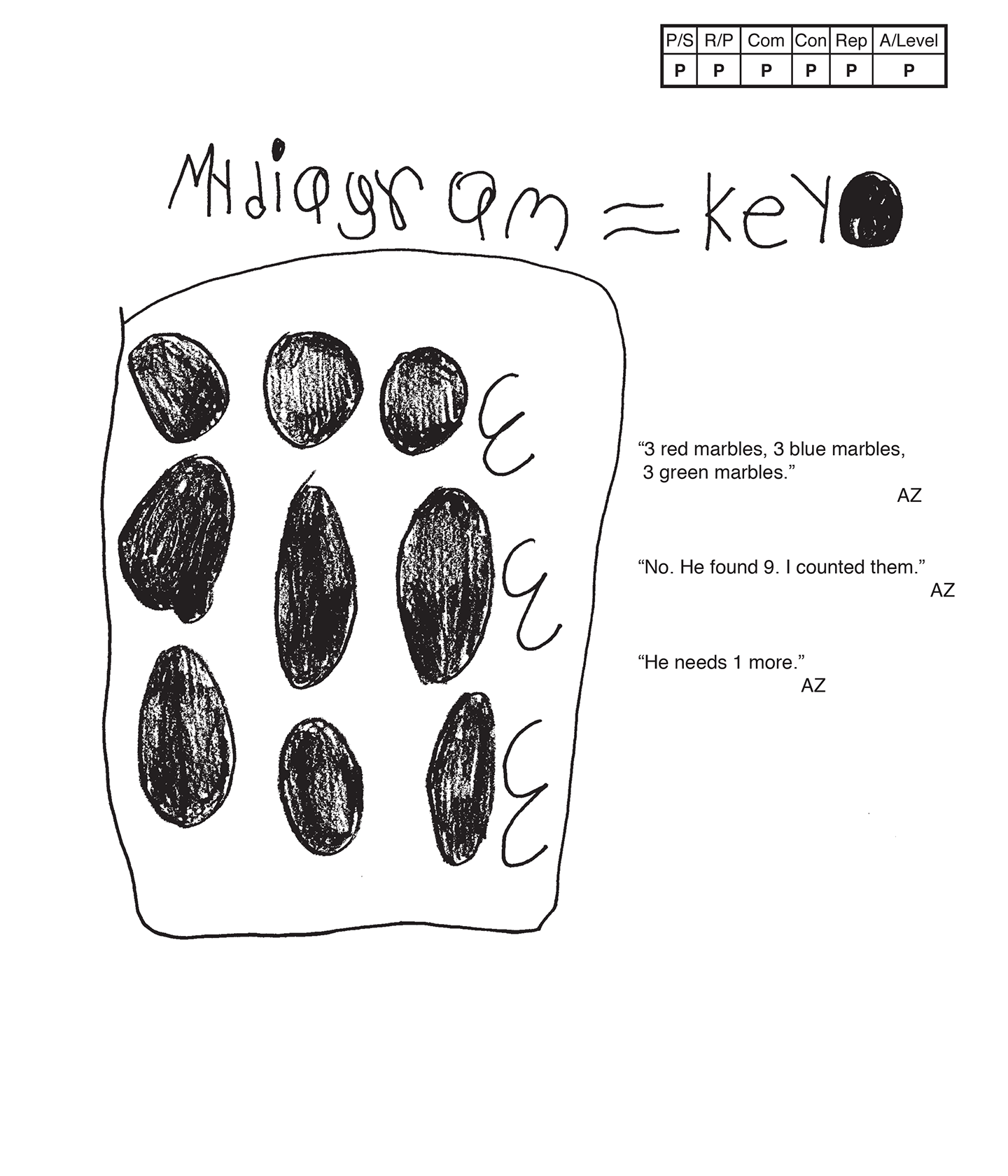This student meets the standard.

### Scoring Rationale

#### Practitioner

The student's strategy of diagramming three red marbles, three blue marbles, and three green marbles for a total of nine marbles and comparing to 10 marbles works to solve this task. The student's answer, "No. He found 9. I counted them," is correct.

#### Practitioner

The student has a systematic approach and provides adequate mathematical basis. The student's diagram and scribing clearly supports the underlying mathematical concept of comparing two totals.

#### Practitioner

The student correctly uses the mathematical terms diagram, more. The student does not earn credit for the term key. The student uses the correct colors in their diagram but uses a pencil-filled-in circle for a marble in the key. The student needs to indicate each color marble in the key.

#### Practitioner

The student makes the mathematically relevant observation, "He needs 1 more."

#### Practitioner

The student's diagram is appropriate to the task and accurate. The scribing provides the necessary labels for each color marble.

#### Practitioner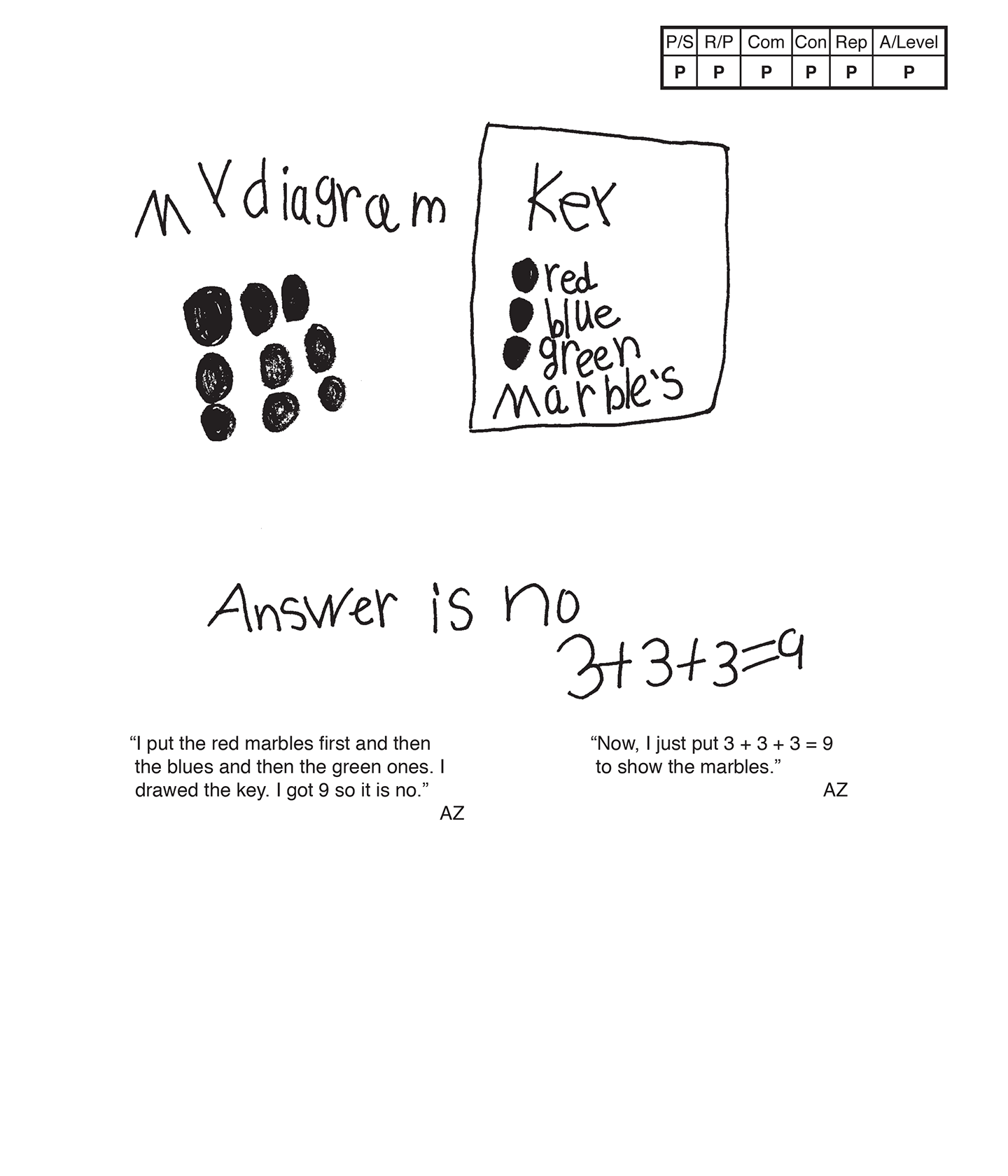This student meets the standard.

### Scoring Rationale

#### Practitioner

The student's strategy of diagramming three red marbles, three blue marbles, and three green marbles for a total of nine marbles works to solve this task. The student's answer, "no," is correct.

#### Practitioner

The student has a systematic approach and provides adequate mathematical basis. The student's diagram and scribing clearly supports the underlying mathematics of comparing two totals.

#### Practitioner

The student correctly uses the mathematical terms diagram, key.

#### Practitioner

The student solves the problem and makes a number sentence, "3 + 3 + 3 = 9." The student does not earn credit for Expert (verification) because the student does not compare the number sentence total to the diagram total stating that they are correct.

#### Practitioner

The student's diagram is appropriate to the task and accurate. The student's key is accurate and the scribing provides the necessary labels for each marble color.

#### Practitioner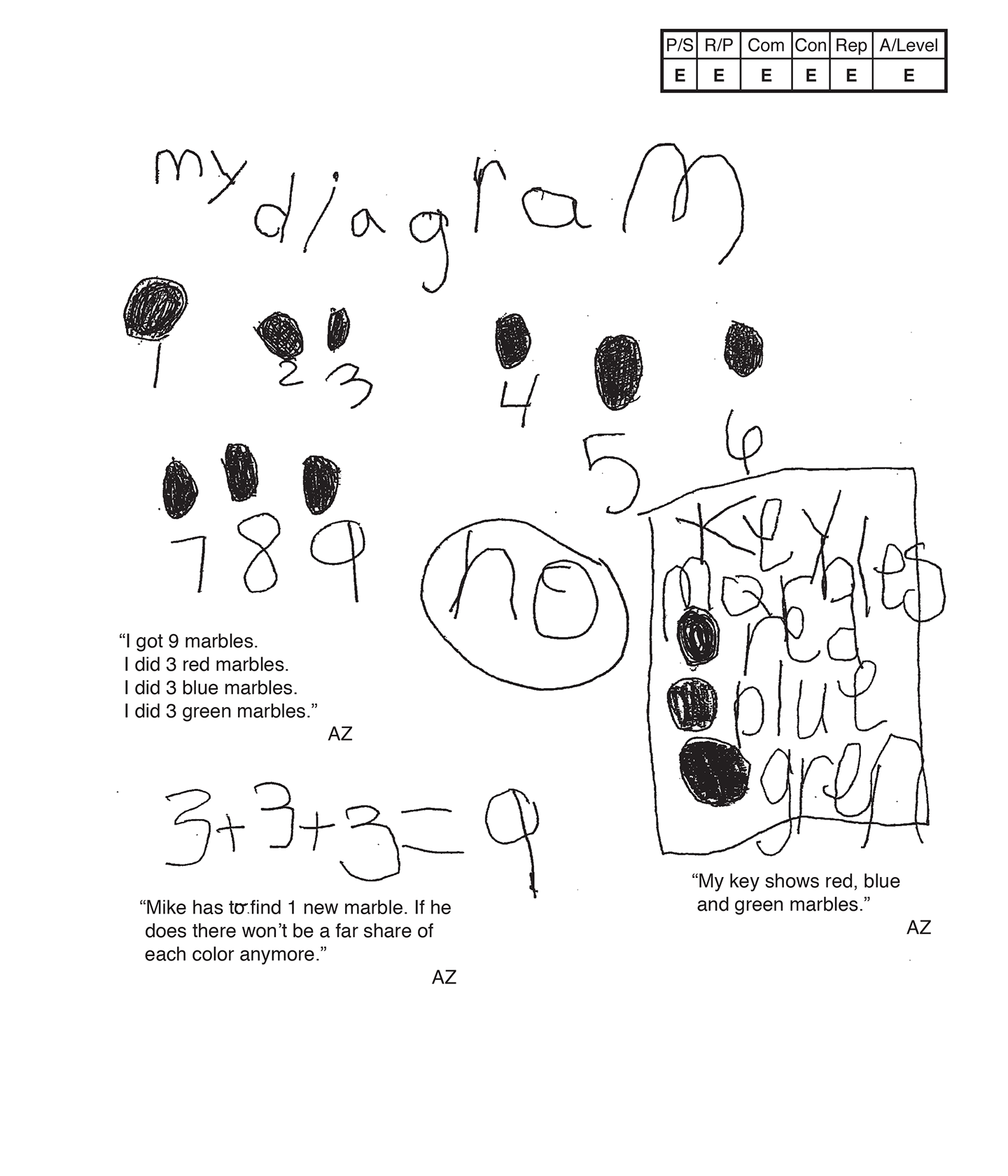,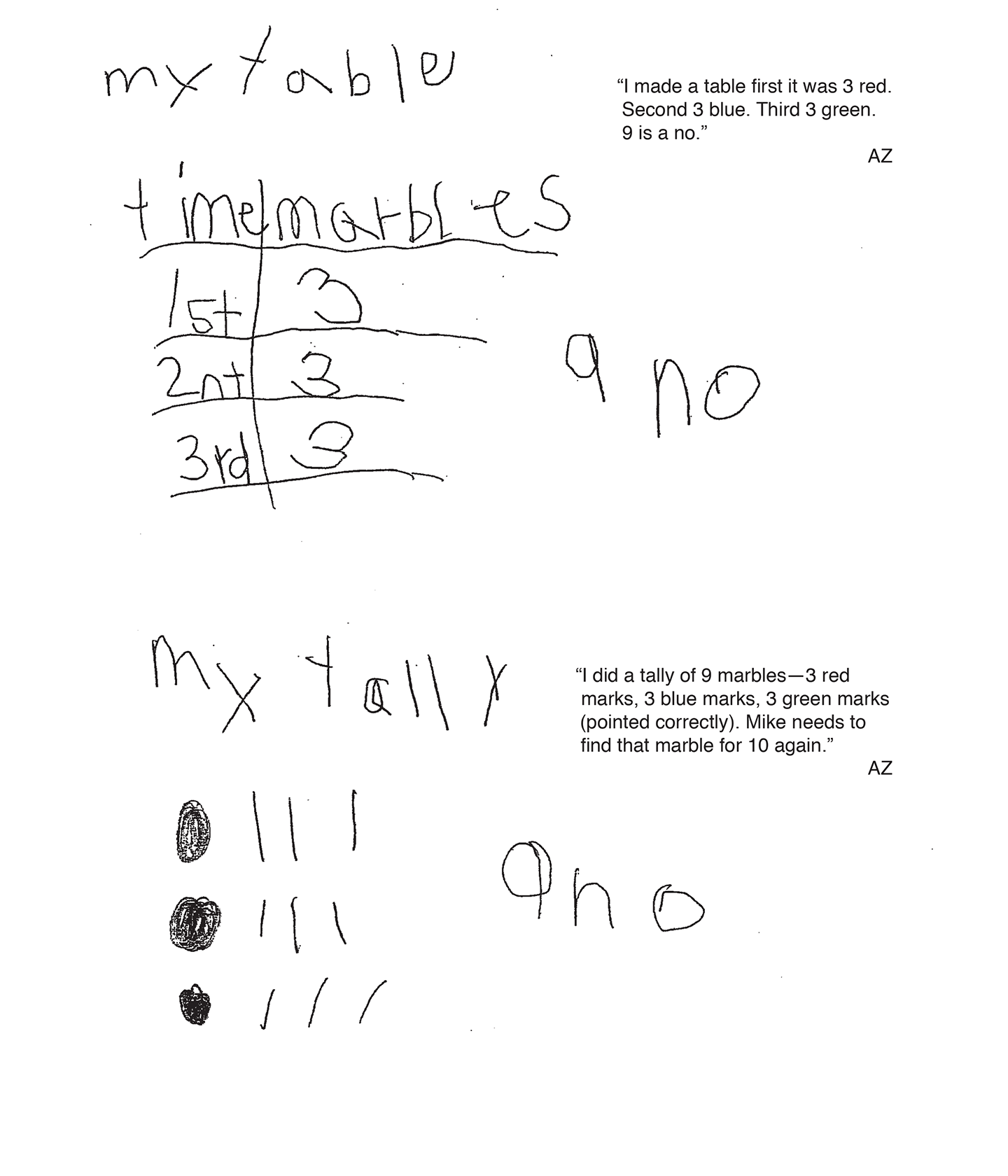This student exceeds the standard.

### Scoring Rationale

#### Expert

The student's strategy of creating a diagram of three red marbles, three blue marbles, and three green marbles for a total of nine marbles and comparing to 10 marbles works to solve this task. The student's answer, "no," is correct. The student uses alternative strategies to solve the same task as well.

#### Expert

The student has a systematic approach and provides adequate mathematical basis. The student justifies and supports the decisions made to arrive at an answer of "no" by the scribing and by using other mathematical strategies.

#### Expert

The student correctly uses the mathematical terms diagram, key, table, first, fair share, second, third, tally.

#### Expert

The student makes the mathematically relevant Practitioner observation, "Mike has to find 1 new marble," and "If he does there won't be a fair share of each color anymore." The student then makes the Expert connection of using other strategies to verify if the answer is correct. The student makes an equation, table, and tally. The student writes "no" beside the table and tally and scribes, "Mike needs to find that marble 10."

#### Expert

The student's diagram is appropriate and accurate. The key and scribing provide the necessary labels for the colored marbles. The student's table is appropriate and accurate with all necessary labels. The student's tally is also appropriate and accurate. The student does not need a key to indicate that one tally mark references one marble. A key is needed only if a tally mark references more than one.